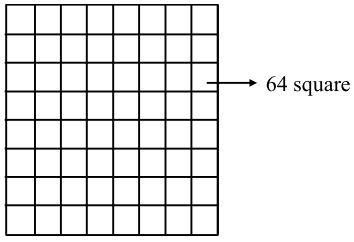# Two squares are chosen`
Question:

Two squares are chosen at random on a chessboard (see figure). The probability that they have a side in common is.1. $\frac{2}{7}$

2. $\frac{1}{18}$

3. $\frac{1}{7}$

4. $\frac{1}{9}$

Correct Option: , 2

Solution:

Total ways of choosing square $={ }^{64} \mathrm{C}_{2}$

$=\frac{64 \times 63}{2 \times 1}=32 \times 63$

ways of choosing two squares having common side $=2(7 \times 8)=112$

Required probability $=\frac{112}{32 \times 63}=\frac{16}{32 \times 9}=\frac{1}{18}$